# An Introduction to DataFrameLast month, we announced .NET support for Jupyter notebooks, and showed how to use them to work with .NET for Apache Spark and ML.NET. Today, we’re announcing the preview of a DataFrame type for .NET to make data exploration easy. If you’ve used Python to manipulate data in notebooks, you’ll already be familiar with the concept of a DataFrame. At a high level, it is an in-memory representation of structured data. In this blog post, I’m going to give an overview of this new type and how you can use it from Jupyter notebooks. To play along, fire up a .NET Jupyter Notebook in a browser.

## How to use DataFrame?

`DataFrame` stores data as a collection of columns. Let’s populate a `DataFrame` with some sample data and go over the major features. The full sample can be found on Github(C# and F#). To follow along in your browser, click here and navigate to csharp/Samples/DataFrame-Getting Started.ipynb(or fsharp/Samples/DataFrame-Getting Started.ipynb). To get started, let’s import the Microsoft.Data.Analysis package and namespace into our .NET Jupyter Notebook (make sure you’re using the C# or F# kernel):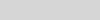Let’s make three columns to hold values of types `DateTime`, `int` and `string`.

``````PrimitiveDataFrameColumn<DateTime> dateTimes = new PrimitiveDataFrameColumn<DateTime>("DateTimes"); // Default length is 0.
PrimitiveDataFrameColumn<int> ints = new PrimitiveDataFrameColumn<int>("Ints", 3); // Makes a column of length 3. Filled with nulls initially
StringDataFrameColumn strings = new StringDataFrameColumn("Strings", 3); // Makes a column of length 3. Filled with nulls initially
``````

`PrimitiveDataFrameColumn` is a generic column that can hold primitive types such as `int`, `float`, `decimal` etc. A `StringDataFrameColumn` is a specialized column that holds `string` values. Both the column types can take a `length` parameter in their contructors and are filled with `null` values initially. Before we can add these columns to a `DataFrame` though, we need to append three values to our `dateTimes` column. This is because the `DataFrame` constructor expects all its columns to have the same length.

``````// Append 3 values to dateTimes
dateTimes.Append(DateTime.Parse("2019/01/01"));
dateTimes.Append(DateTime.Parse("2019/01/01"));
dateTimes.Append(DateTime.Parse("2019/01/02"));
``````

Now we’re ready to create a `DataFrame` with three columns.

``````DataFrame df = new DataFrame(dateTimes, ints, strings); // This will throw if the columns are of different lengths
``````

One of the benefits of using a notebook for data exploration is the interactive REPL. We can enter `df` into a new cell and run it to see what data it contains. For the rest of this post, we’ll work in a .NET Jupyter environment. All the sample code will work in a regular console app as well though.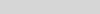We immediately see that the formatting of the output can be improved. Each column is printed as an array of values and we don’t see the names of the columns. If `df` had more rows and columns, the output would be hard to read. Fortunately, in a Jupyter environment, we can write custom formatters for types. Let’s write a formatter for `DataFrame`.

``````using Microsoft.AspNetCore.Html;
Formatter<DataFrame>.Register((df, writer) =>
{
var rows = new List<List<IHtmlContent>>();
var take = 20;
for (var i = 0; i < Math.Min(take, df.Rows.Count); i++)
{
var cells = new List<IHtmlContent>();
foreach (var obj in df.Rows[i])
{
}
}

var t = table(
tbody(
rows.Select(
r => tr(r))));

writer.Write(t);
}, "text/html");
``````

This snippet of code register a new `DataFrame` formatter. All subsequent evaluations of `df` in a notebook will now output the first 20 rows of a `DataFrame` along with the column names. In the future, the `DataFrame` type and other libraries that target Jupyter as one of their environments will be able to ship with their formatters.Sure enough, when we re-evaluate `df`, we see that it contains the three columns we created previously. The formatting makes it much easier to inspect our values. There’s also a helpful `index` column in the output to quickly see which row we’re looking at. Let’s modify our data by indexing into `df`:

``````df[0, 1] = 10; // 0 is the rowIndex, and 1 is the columnIndex. This sets the 0th value in the Ints columns to 10
``````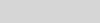We can also modify the values in the columns through indexers defined on `PrimitiveDataFrameColumn` and `StringDataFrameColumn`:

``````// Modify ints and strings columns by indexing
ints = 100;
strings = "Foo!";
``````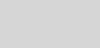One caveat to keep in mind here is the data type of the value passed in to the indexers. We passed in the right data types to the column indexers in our sample: an integer value of `100` to `ints` and a string `"Foo!"` to `string`. If the data types don’t match, an exception will be thrown. For cases where the type of data in the columns is not obvious, there is a handy `DataType` property defined on each column. The `Info` method displays the `DataType` and `Length` properties of each column: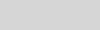The `DataFrame` and `DataFrameColumn` classes expose a number of useful APIs: binary operations, computations, joins, merges, handling missing values and more. Let’s look at some of them:

``````// Add 5 to Ints through the DataFrame
``````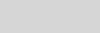``````// We can also use binary operators. Binary operators produce a copy, so assign it back to our Ints column
df["Ints"] = (ints / 5) * 100;
``````All binary operators are backed by functions that produces a copy by default. The `+` operator, for example, calls the `Add` method and passes in `false` for the `inPlace` parameter. This lets us elegantly manipulate data using operators without worrying about modifying our existing values. For when in place semantics are desired, we can set the `inPlace` parameter to `true` in the binary functions.

In our sample, `df` has `null` values in its columns. `DataFrame` and `DataFrameColumn` offer an API to fill `nulls` with values.

``````df["Ints"].FillNulls(-1, inPlace: true);
df["Strings"].FillNulls("Bar", inPlace: true);
``````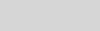`DataFrame` exposes a `Columns` property that we can enumerate over to access our columns and a `Rows` property to access our rows. We can index `Rows` to access each row. Here’s an example that accesses the first row:

``````DataFrameRow row0 = df.Rows;
``````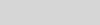To inspect our values better, let’s write a formatter for `DataFrameRow` that displays values in a single line.

``````using Microsoft.AspNetCore.Html;
Formatter<DataFrameRow>.Register((dataFrameRow, writer) =>
{
var cells = new List<IHtmlContent>();
foreach (var obj in dataFrameRow)
{
}

var t = table(
tbody(
cells));

writer.Write(t);
}, "text/html");
``````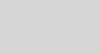To enumerate over all the rows in a `DataFrame`, we can write a simple for loop. `DataFrame.Rows.Count` returns the number of rows in a `DataFrame` and we can use the loop index to access each row.

``````for (long i = 0; i < df.Rows.Count; i++)
{
DataFrameRow row = df.Rows[i];
}
``````

Note that each row is a view of the values in the `DataFrame`. Modifying the values in the `row` object modifies the values in the `DataFrame`. We do however lose type information on the returned `row` object. This is a consequence of `DataFrame` being a loosely typed data structure.

Let’s wrap up our `DataFrame` API tour by looking at the `Filter`, `Sort`, `GroupBy` methods:

``````// Filter rows based on equality
PrimitiveDataFrameColumn<bool> boolFilter = df["Strings"].ElementwiseEquals("Bar");
DataFrame filtered = df.Filter(boolFilter);
``````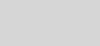`ElementwiseEquals` returns a `PrimitiveDataFrameColumn<bool>` filled with a `true` for every row that equals `"Bar"` in the `Strings` column, and a `false` when it doesn’t equal `"Bar"`. In the `df.Filter` call, each row corresponding to a `true` value in `boolFilter` selects a row out of `df`. The resulting `DataFrame` contains only these rows.

``````// Sort our dataframe using the Ints column
DataFrame sorted = df.Sort("Ints");
// GroupBy
GroupBy groupBy = df.GroupBy("DateTimes");
``````The `GroupBy` method takes in the name of a column and creates groups based on unique values in the column. In our sample, the `DateTimes` column has two unique values, so we expect one group to be created for `2019-01-01 00:00:00Z` and one for `2019-01-02 00:00:00Z`.

``````// Count of values in each group
DataFrame groupCounts = groupBy.Count();
// Alternatively find the sum of the values in each group in Ints
DataFrame intGroupSum = groupBy.Sum("Ints");
``````The `GroupBy` object exposes a set of methods that can called on each group. Some examples are `Max()`, `Min()`, `Count()` etc. The `Count()` method counts the number of values in each group and return them in a new `DataFrame`. The `Sum("Ints")` method sums up the values in each group.

Finally, when we want to work with existing datasets, `DataFrame` exposes a `LoadCsv` method.

``````DataFrame csvDataFrame = DataFrame.LoadCsv("path/to/file.csv");
``````

## Charting

Another cool feature of using a `DataFrame` in a .NET Jupyter environment is charting. XPlot.Plotly is one option to render charts. We can import the `XPlot.Plotly` namespace into our notebook and create interactive visualizations of the data in our `DataFrame`. Let’s populate a `PrimitiveDataFrameColumn<double>` with a normal distribution and plot a histogram of the samples:

``````#r "nuget:MathNet.Numerics,4.9.0"
using XPlot.Plotly;
using System.Linq;
using MathNet.Numerics.Distributions;

double mean = 0;
double stdDev = 0.1;
MathNet.Numerics.Distributions.Normal normalDist = new Normal(mean, stdDev);

PrimitiveDataFrameColumn<double> doubles = new PrimitiveDataFrameColumn<double>("Normal Distribution", normalDist.Samples().Take(1000));
display(Chart.Plot(
new Graph.Histogram()
{
x = doubles,
nbinsx = 30
}
));
``````We first create a `PrimitiveDataFrameColumn<double>` by drawing 1000 samples from a normal distribution and then plot a histogram with 30 bins. The resulting chart is interactive! Hovering over the chart reveals the underlying data and lets us inspect each value precisely.

## Summary

We’ve only explored a subset of the features that `DataFrame` exposes. `Append`, `Join`, `Merge`, and `Aggregations` are supported. Each column also implements `IEnumerable<T?>`, so users can write LINQ queries on columns. The custom `DataFrame` formatting code we wrote has a simple example. The complete source code(and documentation) for `Microsoft.Data.Analysis` lives on GitHub. In a follow up post, I’ll go over how to use `DataFrame` with ML.NET and .NET for Spark. The decision to use column major backing stores (the Arrow format in particular) allows for zero-copy in .NET for Spark User Defined Functions (UDFs)!

We always welcome the community’s feedback! In fact, please feel free to contribute to the source code. We’ve made it easy for users to create new column types that derive from `DataFrameColumn` to add new functionality. Support for structs such as `DateTime` and user defined structs is also not as complete as primitive types such as `int`, `float` etc. We believe this preview package allows the community to do data analysis in .NET. Try out DataFrame in a .NET Jupyter Notebook and let us know what you think!

•Simon Felix

Interesting direction!

Can you elaborate why we need Append, Join, Merge, etc. when there’s LINQ? Are there any advantages to these new way of doing things?
Similarly, what’s the advantage over a collection of mutable, typed objects, structs or anonymous structs? When should I chose which? Why is data exploration easier with the new DataFrame type?

•A number of reasons. For ex: Merge does not exist in LINQ. In general, the APIs on DataFrame that are similar to the ones in LINQ return a DataFrame and do internal book keeping (number of nulls in a column for ex), so they offer more control. I tend to use LINQ to perform the occasional query on a DataFrame, not so much to build a DataFrame itself.

The advantage is that the DataFrame knows how to perform filter, sort, merge etc on all it’s columns. With regular collections of mutable types, you’d likely have to roll out your own implementation that operated on all your collections and kept them in sync. Think about reading a csv file for instance, cleaning up null/missing values and filtering it. I would use a DataFrame here because a) DataFrame already has these APIs implemented and b) My code would still work if I read in a different csv file. With collections of typed objects, each csv file will likely need changes to my code.

IMO the combination of Notebook + DataFrame + Charting is what makes data exploration easy. The notebook lets me see my results and charts at any time and DataFrame lets me manipulate my data however I want. For ex: We have another sample notebook here. You can run that on your machine and go through the exact same steps that someone else went through and look at the results at each step.

•Robert Schmidt

I’m assuming Dataframe support in .NET will cover interoperability with Python. Could you elaborate a bit on this? When will we be able to mix, say, .NET and Python code in the same notebook (by selecting a kernel per cell), and have variables and dataframes flow between them? This would allow each platform to play to their strengths.

•Yup. DataFrame uses the Apache Arrow format as its backing store, so any Arrow formatted data could be wrapped in a DataFrame. On the other side, a DataFrame can also return its data in the Arrow format for something else to consume.

For the second question, I recommend opening an issue here. The notebook team would be the best placed to answer that.

•Andreas Ågren

Interesting! Did you look anything at Deedle? https://fslab.org/Deedle/

•We most definitely did! The XPlot library is from fslab!

•Рихард Дойче

I’m interested in conversion: DataFrame to DataTable and vice versa.
There are a bunch of apis using DataTable (such as odbc drivers).
How to efficiently insert DataFrame to database table?

•Do you mean to replace `DataTable` with `DataFrame`? I would be careful about that. `DataTable` has change tracking and a real connection to a database. For ex: You can update a database with `DataTable`. `DataFrame` just holds values in memory. If you however wanted to go between `DataFrame` and `DataTable` so you can use particular APIs, it should be easy to add constructors and `ToDataTable` methods on `DataFrame`. Maybe open an issue on corefxlab about this?

•Nekketsu Nekketsu

Shouldn’t it use default values?
If we use int -> 0
If we use int? -> null

Same for string and other types.

•Charles Roddie

Getting values by accident without specifying them doesn’t sound like a good idea. Explicit is better.

•Stanton, Andrew

The defaults for types are what is expected. I dont see how that would be considered “by accident”.

•Excellent work!
I was very excited to see these features available in .Net but they do NOT seem to be available for VB.Net.
Could you elaborate on plans to support VB.Net (the .Net language with closest syntax to Python and widely used in data-related projects)?
Would we be able to reference and fully use Microsoft.Data.Analysis from a VB.Net project? I’d appreciate an example for the above article.

•Charles Roddie

Are you sure? The code there looks like very portable, standard .Net code, with a simple well-designed API. So it would be very strage if it did not work in any .Net language.

•Those were my first thoughts, too.
Nevertheless, I hit a roadblock when trying to convert things like this C# line into VB.Net:
PrimitiveDataFrameColumn ints = new PrimitiveDataFrameColumn(“Ints”, 3);

I unsuccessfully tried:
Dim ints As PrimitiveDataFrameColumn(Of Integer) = New PrimitiveDataFrameColumn(Of Integer)(“Ints”, 3)
and
Dim ints As PrimitiveDataFrameColumn(Of Integer?) = New PrimitiveDataFrameColumn(Of Integer?)(“Ints”, 3)

I do have the assembly reference to Microsoft.Data.Analysis and the Imports line for it and they work fine.

Any suggestions would be highly appreciated!

•Hieronymus, Seth

Unfortunately the F# demo is broken for me. It returns the following error:

File Load Error for DataFrame-Getting Started.ipynb
Unreadable Notebook: /home/jovyan/Notebooks/fsharp/Samples/DataFrame-Getting Started.ipynb NotJSONError(‘Notebook does not appear to be JSON: \'{\n “cells”: [\n {\n “cell_type”: “m…’)

Looking at GitHub (https://github.com/dotnet/try/blob/master/NotebookExamples/fsharp/Samples/DataFrame-Getting%20Started.ipynb), there was a recent merge. Could that have broken the format?

EDIT: Looks like an extra trailing comma on line 70 in this commit:
https://github.com/dotnet/try/commit/894901a8b29f8e5300a7f5efe6b72452fb4d24e2

Thanks.

•Thanks Seth! You are indeed right. I put up a fix for it here. Once the fix is merged, the sample should work 🙂

•Hieronymus, Seth

Thanks for fixing it.
Seth

•Mikael Öhman

Excellent. Really appreciate the work here.

Sad to see Deedle be superseeded but so long as this feature is given enough attention I am happy.

From the context of data science in finance, we would like feature equality with pandas, especially time series operations such as resampling, winsorizing extreme quantiles and more.

XPlot with plotly is great but we still need something lika seaborn/matplotlib to produce many non interactive plots.

If thats too much them the interop story w Python should be given high priority.

Its hard to say why dataframes and tidy data make such a huge difference for data science, but they really do.

•Hey Mikael,

Thanks for your support. Do you have examples of things you’d like to do with DataFrame? We haven’t implemented resampling yet(you can write an extension method for it though), but you can use Math.NET to calculate quantiles(each column is exposed as an `IEnumerable<T>`) and `DataFrameColumn.Clip` to clip values.

Are you looking for something like `dataFrame.plot(kind="bar", x=xColumn, y=yColumn)` to produce a bar chart for example? If so, we’ve had discussions before about have an extensions package that implements `plot`, handles legends etc and wraps Plotly(or another backend). It could be community driven too.

•Mikael Öhman

I’ve had time to check some of the APIs now and this is my feedback. Please tell me if it would be valuable to put it in one or more issues on github. Sorry if I have missed something that already exists. I just tested this yesterday so please forgive any mistakes.

Before saying anything else, I would just like to say that I think this whole initiative is awesome! Great job.

Is there an F# tailored interface?

I didn’t see one and while it’s possible to use all features the differences between F# and C# really stand out for some operations. Example (grid approximation of posterior distribution):

“`F#
let l2 = ps1 |> Array.map (fun p -> Binomial.PMFLn(p, n2, k2))
let p2 = ps1 |> Array.map (fun p -> ContinuousUniform.PDFLn(0., 1., p))
let l2Col = PrimitiveDataFrameColumn(“Likelihood”, l2)
let p2Col = PrimitiveDataFrameColumn(“Prior”, p2)
let bdf2 = DataFrame(l2Col, p2Col)

// The unstandardized likelihood.
bdf2.[“UnstdPostLn”] <- bdf2.[“Likelihood”] + bdf2.[“Prior”]

// What I really want to do is the equivalent of pandas “assign” operation. I want to create a new column based on existing columns
// in a non-trivial way. The only alternative I found was to clone and then apply elementwise.
bdf2.[“UnstdPost”] <- bdf2.[“UnstdPostLn”].Clone()

// Here, type information is lost so I have to cast. Then I have to work with nullable which is a pain.
// F# has good support for a lot of nullable operators but no support for when you want to apply functions like exp.
(bdf2.[“UnstdPost”] :?> PrimitiveDataFrameColumn).ApplyElementwise(fun (x: Nullable) i -> Nullable(exp x.Value))

// Normalizing constant
let evidence2 = bdf2.[“UnstdPost”].Sum() :?> float
bdf2.[“StdPostLn”] <- bdf2.[“UnstdPost”] – log evidence2

// Final, standardized posterior approximation. Same issues as before.
bdf2.[“StdPost”] PrimitiveDataFrameColumn).ApplyElementwise(fun x i -> Nullable(exp x.Value))

“`

I don’t think this code is that nice from an F# perspective. I would hope that some of these quirks can be done away with either by tailoring an interface for F# or by making some other adjustments, discussed below.

Extend the concept of an index

In other dataframe solutions the concept of an index column takes a central role. Usually this is an integer or a datetime. This then enables easy joins and with new timeseries and other operations. One example for timeseries data is resampling. That is, given data on a millisecond basis I may want to resample that data to seconds and perform a custom aggregation in doing so (see pandas resample).

In the NET implementation, there is an index but it’s always integer based and you can’t supply it when creating a series (data frame column). This makes it harder than would have to be to quickly put together a dataframe from disparate data sources. Requiring the length
of all columns to be the same is not good enough for production use and inhibits productivity.

See pandas or deedle:

Missing values treatment

On the dataframe there is a `DropNulls` operation but not when working on individual columns?

From my previous code example, what I could have been OK with would have been to drop all nulls from the column and then call `Apply` with my custom function, not having to deal with `Nullable`. This would have given me a new column where I have my index info (datetime) together with my new values. Then I would assign that to a new column in my dataframe. For the indices where I am missing values, the dataframe would just know that.

Currently that’s not possible (?) and it makes anything non-trivial a hassle.

Time series operations

The dataframe comes from the world of time series analysis in different forms. I think the design and implementation should recognize and honour that. Otherwise I don’t see the point as that’s where practically all applications lie.

This means out-of-the-box support for standard calculations such as moving averages. Much of this can of course be done in a third-party library but at least the necessary concepts have to exist. As I see it this is primarily what’s called “window”-functionality. In deedle and pandas it’s possible to perform windowed calculations. Either a moving window of a fixed size or an expanding window that adds a new row for each iteration. This is really useful for smoothing data and the expanding functionality is very powerful for making sure that all computations are done in a chronologically consistent way (no benefit of hindsight).

See pandas or deedle:

windowing

Summary

Great initiative. Please improve F# experience, introduce concept of an index (usually datetime-based) and put time series analysis in center-stage.

•Mikael Öhman

As you say I think plot libs should mostly be community driven. But what Microsoft could do is make it easy for us to utilize other backends existing in python and R. Primarily I’m thinking of matplotlib and ggplot.

I don’t know exactly how that’s best accomplished.

I know that in Jupyter right now it is possible to interop between R and py using some libs. For example I can pass a pandas dataframe to R and plot with ggplot. If NET can have the same type of interop then anything we can’t do in NET plot libs we can do in ggplot or matplotlib.

The problem with Plotly as I see it is that everything is interactive. That’s great sometimes, but sometimes you just want a facade of 20-30 plots side by side in which case interaction means your browser will consume all your memory and Jupyter will not work.

•Stanton, Andrew

I’ve used DataFrame before in python, and explained it to others as “Its like dataset/table” in .NET. I’m not sure what the point of this port is when there is already a corollary present in .NET (thats had a Merge operation since forever, and can be Typed or Untyped). What is the benefit of using this new thing over a well tested existing thing?

•This is the first question we considered! Take a look at the discussion here: https://github.com/dotnet/corefx/issues/26845.

•Filippo Rottoli

It looks all great!
Sorry, just a couple of questions: is there any plan to integrate the .NET Notebooks with the SciSharp Stack (e.g. with NumSharp, Pandas.NET, scikit-learn.NET or Matplotlib.NET)? In particular, will the DataFrame object described in this post be in some way compliant (or convertible to) that used in Pandas.NET?
In addition, I am curious to know in the future the answer to the question asked by Robert Schmidt (“When will we be able to mix, say, .NET and Python code in the same notebook (by selecting a kernel per cell), and have variables and dataframes flow between them? This would allow each platform to play to their strengths.”). This looks very similar to me to what is in progress with Polynote polyglot project, which I think it’s awesome!

•There are no plans I’m aware of to integrate with SciSharp. However, given that each column exposes it’s data as an `IEnumerable<T>`, I would expect that we can use NumSharp/Scikit-learn.NET. I haven’t looked into how SciSharp stores it’s data yet, but unless they use the Apache Arrow format, there’ll likely be a penalty to go from `DataFrame` to `Pandas.NET` or vice-versa. What `DataFrame` does do though is integrate with ML.NET, .NET for Spark and anything else that can consume Arrow formatted data.

Unfortunately, I don’t know enough about the notebook team’s plans yet for the polyglot scenario. But, if you opened an issue here, you could hear from folks working on the notebook experience itself 🙂

•Filippo Rottoli